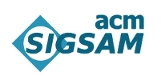# Computer Algebra Software

SIGSAM maintains this collection of references to computer algebra systems, to support our citation policy. Click the name of each system to see further information, links and a citation in BibTeX format. If you have suggestions for changes or additions to this list please contact Infodir_SIGSAM@acm.org.

## Broad purpose free computer algebra systems

• Axiom: a general-purpose, strongly typed, computer algebra system.
• CoCoA: a computer algebra system for doing computations in Commutative Algebra.
• Fermat: a computer algebra system oriented towards polynomial and matrix algebra over the rationals and finite fields.
• GAP: a System for Computational Discrete Algebra.
• KASH/KANT: computer algebra system for sophisticated computations in algebraic number fields and global function fields.
• Macaulay2: a system for research in algebraic geometry and commutative algebra.
• Maxima: Maxima is an active open source project or the manipulation of symbolic and numerical expressions.
• Reduce: an interactive system for general algebraic computations of interest to mathematicians, scientists and engineers.
• SageMath: an open-source general purpose computer algebra system.
• SINGULAR: a Computer Algebra System for polynomial computations with special emphasis on the needs of commutative algebra, algebraic geometry, and singularity theory.
• PARI/GP: a computer algebra system designed for for fast computations in number theory.

## Special Purpose Systems, Packages and Libraries

• ACE : a Maple library providing tools useful in algebraic combinatorics.
• Albert: an interactive program to assist the specialist in the study of nonassociative algebras.
• ANUNQ: a GAP package for the computation of nilpotent factor groups of finitely presented groups.
• ANUPQ: an interactive interface to the p-quotient, p-group generation and standard presentation algorithms of the ANU pq C program.
• CALI: a REDUCE package for computational commutative algebra.
• CASA: a Computer Algebra System for Algebraic Geometry.
• CHEVIE: a computer algebra system for symbolic calculations with generic character tables of groups.
• EinS: a Mathematica package allowing one to perform symbolic calculations with indexed objects.
• Felix: a special computer algebra system for the computation in commutative and non-commutative rings and modules.
• FeynArts: a Mathematica package for the generation and visualization of Feynman diagrams and amplitudes.
• GiNaC: a system to allow the creation of integrated systems that embed symbolic manipulations together with more established areas of computer science.
• GRAPE: a GAP package for constructing and analysing graphs related to groups, finite geometries, and designs.
• GUAVA: a GAP package for computing with error-correcting codes.
• LiDIA: A C++ Library For Computational Number Theory.
• LiE: A Computer algebra package for Lie group computations.
• MOLGEN: a system for the computation of all structural formulae that correspond to a given molecular formula.
• ORME: a package for equational theoreies.
• Rubi: a package for Rule-based Integration.
• SONATA: a system for the construction and the analysis of finite nearrings.

### Volunteers Needed

We are looking for volunteers to contribute content to the website. If you are interested please contact Infodir_SIGSAM@acm.org.

### In Particular

• Could you help update this list of Computer Algebra Software?
• Could you provide some more detailed descriptions?
• Could you contribute an impartial review?